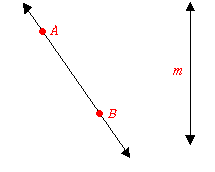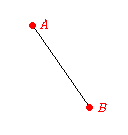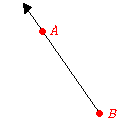# Lines, Segments, and Rays

Although we all know intuitively what a line is, it is actually difficult to give a good mathematical definition. Roughly, we can say that a line is an infinitely thin, infinitely long collection of points extending in two opposite directions. When we draw lines in geometry, we use an arrow at each end to show that it extends infinitely.A line can be named either using two points on the line (for example, $\stackrel{↔}{AB}$ ) or simply by a letter, usually lowercase (for example, line $m$ ).

A line segment has two endpoints. It contains these endpoints and all the points of the line between them. You can measure the length of a segment, but not of a line.A segment is named by its two endpoints, for example, $\stackrel{¯}{AB}$ .

A ray is a part of a line that has one endpoint and goes on infinitely in only one direction. You cannot measure the length of a ray.A ray is named using its endpoint first, and then any other point on the ray (for example, $\stackrel{\to }{BA}$ ).